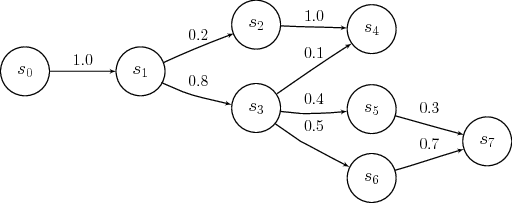# flock-botnet

A twitter botnet with autonomous bots replying tweets with text generated based on probabilities in Markov chains

## How it works

Get a large input text.

### Generate Markov chains

From a large input text, generates the Markov chains calculating the probabilites.

### Each bot process a tweet

Basing on the tweet content, generating the reply using Markov chains.

The bot publishes the generated reply. And starts the process again.

## Markov chain

A Markov chain is Sequence of stochastic events (based on probabilities) where the current state of a variable or system is independent of all past states, except the current state.

## Generating text with Markov chains

The algorithm calculates the probabilities of Markov chains, analyzing a considerable amount of text, for the examples, I've done it with the book "The Critique of Pure Reason", by Immanuel Kant.

Each word is considered as a Markov state, and the algorithm calculates the probabilities to get the next state. After processing a large amount of text, it have a 'memory' with all the possible states of the text with the probabilities to the next states.When the botnet is up working, the bots start streaming all the twitter new tweets containing the configured keywords. Each bot takes a tweet, analyzes the containing words, and generates a reply using the Markov chains previously calculated, and posts the tweet as reply.

## Result

The result is a twitter botnet, controlled by the script. The botnet interact with humans, replying responses generated with the algorithm.

In the examples can see the type of response. For future versions, can be a good point to feed the text database with more books, to get a text database bigger.